Processing ......FreeComputerBooks.com Links to Free Computer, Mathematics, Technical Books all over the World

Seeing Theory: A Visual Introduction to Probability and Statistics
🌠 Top Free Mathematics Books - 100% Free or Open Source!
• Title Seeing Theory: A Visual Introduction to Probability and Statistics
• Author(s) Daniel Kunin, Jingru Guo, Tyler Dae Devlin, Daniel Xiang
• Publisher: Brown University
• Paperback N/A
• eBook PDF
• Language: English
• ISBN-10/ASIN: N/A
• ISBN-13: N/A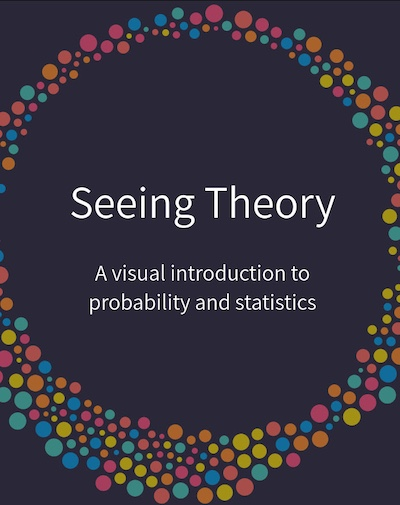Book Description

Seeing Theory was created by Daniel Kunin while an undergraduate at Brown University. The goal of this website is to make statistics more accessible through interactive visualizations (designed using Mike Bostock’s JavaScript library D3.js).

Statistics is quickly becoming the most important and multi-disciplinary field of mathematics. According to the American Statistical Association, statistician is one of the top ten fastest-growing occupations and statistics is one of the fastest-growing bachelor degrees. Statistical literacy is essential to our data driven society.

Despite the increased importance and demand for statistical competence, the pedagogical approaches in statistics have barely changed. Using Mike Bostock's data visualization software, D3.js, Seeing Theory visualizes the fundamental concepts covered in an introductory college statistics or Advanced Placement statistics class. Students are encouraged to use Seeing Theory as an additional resource to their textbook, professor and peers.

The authors believe that it is far more important for a student to develop statistical intuition than to be able to recite equations. They have developed a collection of 15 interactive visualizations, each of which presents a bite-sized concept that is encountered in an introductory statistics curriculum. By using visual explanations, we're able to reach students for whom purely mathematical notation is all but impenetrable. By using interactive visualizations, we encourage students to engage and explore.

• N/A
Reviews, Ratings, and Recommendations: Related Book Categories: Read and Download Links:Similar Books:
•Introduction to Probability, Statistics, and Random Processes

This book introduces students to probability, statistics, and stochastic processes. It provides a clear and intuitive approach to these topics while maintaining mathematical accuracy. The book contains a large number of solved exercises.

•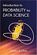Introduction to Probability for Data Science (Stanley Chan)

This book is an introductory textbook in undergraduate probability in the context of data science to emphasize the inseparability between data (computing) and probability (theory) in our time, with examples in both MATLAB and Python.

•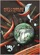Mostly Harmless Statistics (Rachel L. Webb)

This text is for an introductory level probability and statistics course with an intermediate algebra prerequisite. The focus of the text follows the American Statistical Association's Guidelines for Assessment and Instruction in Statistics Education (GAISE).

•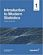Introduction to Modern Statistics (Mine Çetinkaya-Rundel, et al.)

This book puts a heavy emphasis on exploratory data analysis and provides a thorough discussion of simulation-based inference using randomization and bootstrapping, followed by a presentation of the related Central Limit Theorem based approaches.

•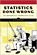Statistics Done Wrong: The Woefully Complete Guide (Reinhart)

Scientific progress depends on good research, and good research needs good statistics. But statistical analysis is tricky to get right, even for the best and brightest of us. You'd be surprised how many scientists are doing it wrong.

•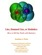Lies, Damned Lies: How to Tell the Truth with Statistics

The goal is to help you learn How to Tell the Truth with Statistics and, therefore, how to tell when others are telling the truth ... or are faking their "news". Covers Data Analysis, Binomial and normal models, Sample statistics, confidence intervals, hypothesis tests, etc.

•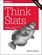Think Stats, 2nd Edition: Exploratory Data Analysis in Python

This concise introduction shows you how to perform statistical analysis computationally, rather than mathematically, with programs written in Python. You'll become familiar with distributions, the rules of probability, visualization, and many other tools and concepts.

•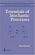Essentials of Stochastic Processes (Rick Durrett)

Stochastic processes have become important for many fields, including mathematical finance and engineering. Written by one of the worlds leading probabilists, this book presents recent results previously available only in specialized monographs.

•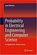Probability in Electrical Engineering and Computer Science

This textbook motivates and illustrates the techniques of applied probability by applications in electrical engineering and computer science (EECS). Python labs enable the readers to experiment and consolidate their understanding.

Book Categories
 :All CategoriesTop Free BooksRecent BooksMiscellaneous BooksComputer EngineeringComputer LanguagesComputer ScienceData Science/DatabasesJava and Java EE (J2EE)Linux and UnixMathematicsMicrosoft and .NETMobile ComputingNetworking and CommunicationsSoftware EngineeringSpecial TopicsWeb Programming
Other Categories